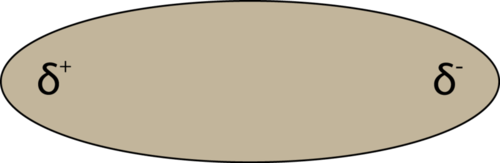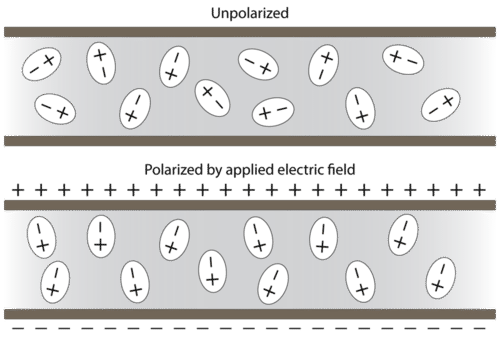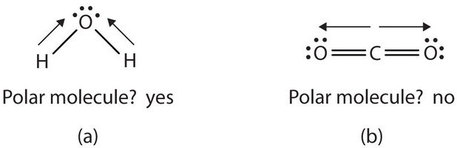# 4.9: Polar Molecules

$$\newcommand{\vecs}{\overset { \rightharpoonup} {\mathbf{#1}} }$$ $$\newcommand{\vecd}{\overset{-\!-\!\rightharpoonup}{\vphantom{a}\smash {#1}}}$$$$\newcommand{\id}{\mathrm{id}}$$ $$\newcommand{\Span}{\mathrm{span}}$$ $$\newcommand{\kernel}{\mathrm{null}\,}$$ $$\newcommand{\range}{\mathrm{range}\,}$$ $$\newcommand{\RealPart}{\mathrm{Re}}$$ $$\newcommand{\ImaginaryPart}{\mathrm{Im}}$$ $$\newcommand{\Argument}{\mathrm{Arg}}$$ $$\newcommand{\norm}{\| #1 \|}$$ $$\newcommand{\inner}{\langle #1, #2 \rangle}$$ $$\newcommand{\Span}{\mathrm{span}}$$ $$\newcommand{\id}{\mathrm{id}}$$ $$\newcommand{\Span}{\mathrm{span}}$$ $$\newcommand{\kernel}{\mathrm{null}\,}$$ $$\newcommand{\range}{\mathrm{range}\,}$$ $$\newcommand{\RealPart}{\mathrm{Re}}$$ $$\newcommand{\ImaginaryPart}{\mathrm{Im}}$$ $$\newcommand{\Argument}{\mathrm{Arg}}$$ $$\newcommand{\norm}{\| #1 \|}$$ $$\newcommand{\inner}{\langle #1, #2 \rangle}$$ $$\newcommand{\Span}{\mathrm{span}}$$

##### Learning Objectives
• Recognize bond characteristics of covalent compounds: bond length and bond polarity.
• Use electronegativity values to predict bond polarity.

## Bond Polarity and Molecular Polarity

If there is only one bond in the molecule, the bond polarity determines the molecular polarity. Any diatomic molecule in which the two atoms are the same element must be a nonpolar molecule. A diatomic molecule that consists of a polar covalent bond, such as HF, is a polar molecule. A polar molecule is a molecule in which one end of the molecule is slightly positive, while the other end is slightly negative. The two electrically charged regions on either end of the molecule are called poles, similar to a magnet having a north and a south pole. Hence, a molecule with two poles is called a dipole. A simplified way to depict polar molecules like HF is pictured below (see figure below).Figure $$\PageIndex{5}$$: A molecular dipole results from the unequal distribution of electron density throughout a molecule.

When placed between oppositely charged plates, polar molecules orient themselves so that their positive ends are closer to the negative plate and their negative ends are closer to the positive plate (see Figure 4.4.6 below).Figure $$\PageIndex{6}$$: Polar molecules are randomly oriented in the absence of an applied electric field (top). In an electric field, the molecules orient themselves to maximize the attraction between opposite charges (bottom).

Experimental techniques involving electric fields can be used to determine if a certain substance is composed of polar molecules and to measure the degree of polarity.

For molecules with more than two atoms, the molecular geometry must also be taken into account when determining if the molecule is polar or nonpolar. (Figure $$\PageIndex{7}$$) is a comparison between carbon dioxide and water. Carbon dioxide (CO2) is a linear molecule. The oxygen atoms are more electronegative than the carbon atom, so there are two individual dipoles pointing outward from the C atom to each O atom. However, since the dipoles are of equal strength and are oriented directly opposite each other, they cancel each other out, and the overall molecular polarity of CO2 is zero. CO2 is a nonpolar molecule. In H2O, the orientation of the two O–H bonds is bent: one end of the molecule has a partial positive charge, and the other end has a partial negative charge. In short, the molecule itself is polar. The polarity of water has an enormous impact on its physical and chemical properties. For example, the boiling point of water (100°C) is high for such a small molecule due to the fact that polar molecules attract each other strongly. On the other hand, the nonpolar carbon dioxide becomes a gas at −77°C, almost 200° lower than the temperature at which water boils. More details will be presented in the next section.Figure $$\PageIndex{7}$$ Physical Properties and Polarity. The physical properties of water and carbon dioxide are affected by their polarities.

## Concept Review Exercises

1. What is the name for the distance between two atoms in a covalent bond?

2. What does the electronegativity of an atom indicate?

3. What type of bond is formed between two atoms if the difference in electronegativities is small? Medium? Large?

1. bond length

2. Electronegativity is a qualitative measure of how much an atom attracts electrons in a covalent bond.

3. nonpolar; polar; ionic

## Key Takeaways

• Covalent bonds between different atoms have different bond lengths.
• Covalent bonds can be polar or nonpolar, depending on the electronegativity difference between the atoms involved.

## Exercises

1. Which is longer—a C–H bond or a C–O bond? (Refer to Table $$\PageIndex{1}$$.)

2. Which is shorter—an N–H bond or a C–H bond? (Refer to Table $$\PageIndex{1}$$.)

3. A nanometer is 10−9 m. Using the data in Table $$\PageIndex{1}$$ and Table $$\PageIndex{2}$$, determine the length of each bond in nanometers.

1. a C–O bond
2. a C=O bond
3. an H–N bond
4. a C≡N bond
4. An angstrom (Å) is defined as 10−10 m. Using Table $$\PageIndex{1}$$ and Table $$\PageIndex{2}$$, determine the length of each bond in angstroms.

1. a C–C bond
2. a C=C bond
3. an N≡N bond
4. an H–O bond
5. Refer to Exercise 3. Why is the nanometer unit useful as a unit for expressing bond lengths?

6. Refer to Exercise 4. Why is the angstrom unit useful as a unit for expressing bond lengths?

7. Using Figure $$\PageIndex{3}$$, determine which atom in each pair has the higher electronegativity.

1. H or C
2. O or Br
3. Na or Rb
4. I or Cl
8. Using Figure $$\PageIndex{3}$$, determine which atom in each pair has the lower electronegativity.

1. Mg or O
2. S or F
3. Al or Ga
4. O or I
9. Will the electrons be shared equally or unequally across each covalent bond? If unequally, to which atom are the electrons more strongly drawn?

1. a C–O bond
2. an F–F bond
3. an S–N bond
4. an I–Cl bond

10. Will the electrons be shared equally or unequally across each covalent bond? If unequally, to which atom are the electrons more strongly drawn?

• a C–C bond
• a S–Cl bond
• an O–H bond
• an H–H bond

11. Arrange the following bonds from least polar to most polar: H-F, H-N, H-O, H-C

12. Arrange the following bonds from least polar to most polar: C-F, C-N, C-O, C-C

1. A C–O bond is longer.

2. An H-N bond is shorter than an H-C bond.
1. 0.143 nm
2. 0.120 nm
3. 0.100 nm
4. 0.116 nm

4.

1. 1.54 Å
2. 1.34 Å
3. 1.10 Å
4. 0.97 Å
1. Actual bond lengths are very small, so the nanometer unit makes the expression of length easier to understand.

6. Actual bond lengths are very small, so the angstrom unit makes the expression of length easier to understand.
1. C
2. O
3. Na
4. Cl
8.
1. Mg
2. S
3. Al
4. I

9.

1. unequally toward the O
2. equally
3. unequally toward the N
4. unequally toward the Cl

10.

• equally
• unequally toward the Cl
• unequally toward the O
• equally

11. The electronegativity difference increases from 0.4; 0.9; 1.4; 1.9. Hence, the least to most polar: H-C, H-N, H-O, H-F

12. The electronegativity difference increases from 0; 0.5; 1.0; 1.5. Hence, the least to most polar: C-C, C-N, C-O, C-F

4.9: Polar Molecules is shared under a not declared license and was authored, remixed, and/or curated by LibreTexts.